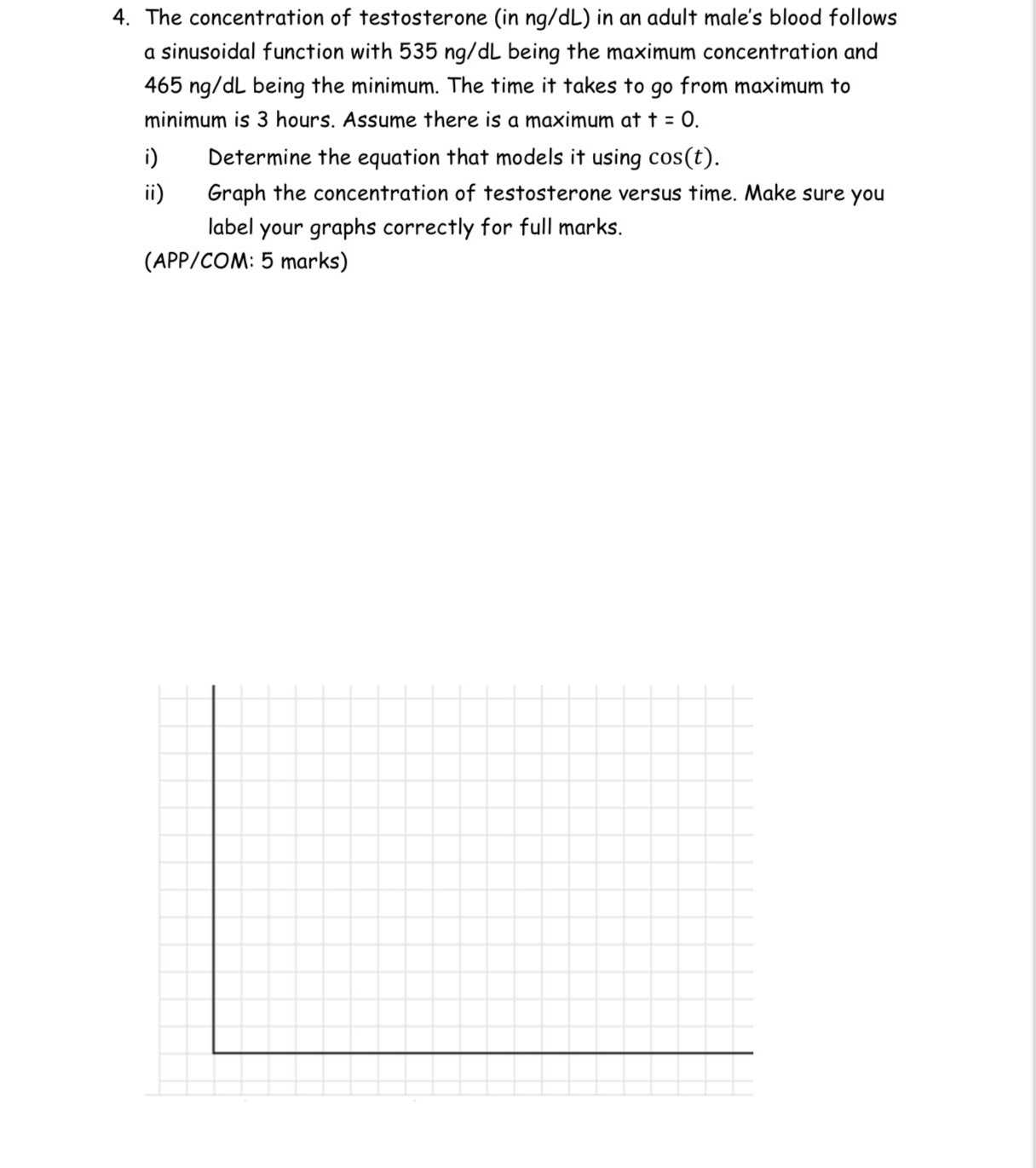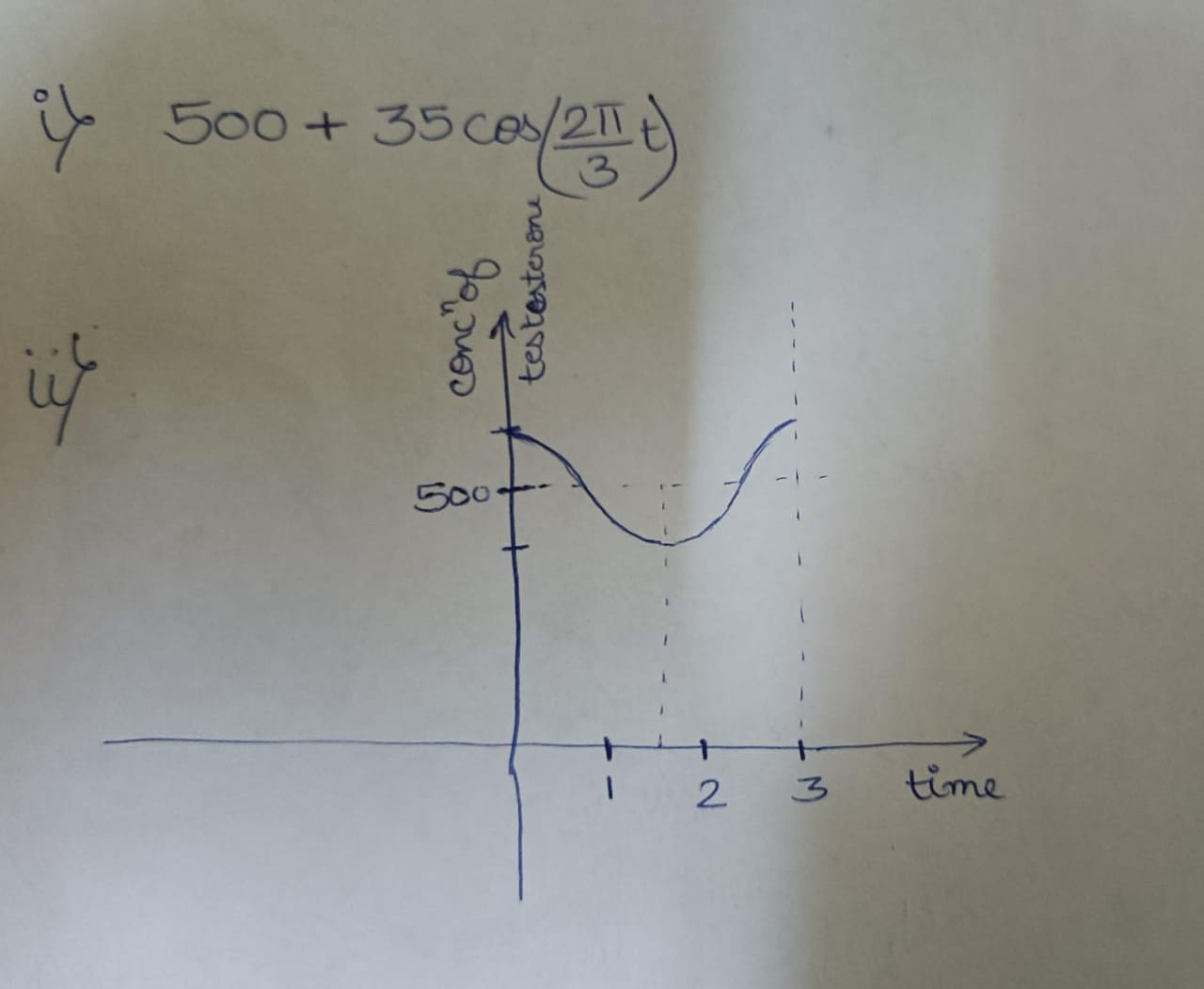### ¿Todavía tienes preguntas de matemáticas?

Pregunte a nuestros tutores expertos
Algebra
Pregunta4. The concentration of testosterone (in $$ng / dL$$ ) in an adult male's blood follows a sinusoidal function with $$535 ng / dL$$ being the maximum concentration and $$465 ng / dL$$ being the minimum. The time it takes to go from maximum to minimum is $$3$$ hours. Assume there is a maximum at $$t = 0$$ .

i) Determine the equation that models it using $$\cos ( t )$$ .

ii) Graph the concentration of testosterone versus time. Make sure you label your graphs correctly for full marks.